# Building VGG and Wide Residual Networks in Keras for Image Classification¶

#### An introduction to rapid network prototyping with Keras.¶

In this tutorial I demonstrate how Keras can be used to quickly implement deep learning architectures for rapid prototyping. Specifically, I build and train two networks on the CIFAR-10 dataset with image augmentation:

1. A simplified VGG architecture using the Keras Sequential API.
2. A Wide Residual Network (WRN) using the Keras Functional API.

Why Keras? In my earlier tutorial I demonstrated how to use the low-level TensorFlow API to build and train a simple convolutional neural network (CNN). But such an approach is code-heavy and quickly becomes cumbersome when trying to rapidly prototype multiple ideas. Keras was designed to eliminate much of this overhead, making it faster to move from idea to experiment.

As well-articulated by Keras creator Francois Chollet in his post on the recent success of Keras in winning Kaggle competitions:

"Your final models will typically share little in common with the solutions you envisioned when first approaching the problem, because ...a-priori plans basically never survive confrontation with experimental reality. So winning is not so much about how good your theoretical vision is, it's about how much contact with reality your vision has been through. ...[Using] Keras allows you to iterate faster, to try more things. Ultimately, this enables you to win competitions (or publish papers). Being able to go from idea to result with the least possible delay is key to doing good research —that’s one of the core beliefs behind Keras."

### Import Libraries¶

In :
import pickle
import os
import math
import numpy as np

from datetime import datetime
from matplotlib import pyplot as plt
from IPython.display import Image

import tensorflow as tf
import keras as K

from keras.layers import Input, Conv2D, Dense
from keras.layers import BatchNormalization, SpatialDropout2D
from keras.layers import Activation, Dropout, MaxPooling2D
from keras.preprocessing.image import ImageDataGenerator
from keras.callbacks import TensorBoard
from keras.callbacks import LearningRateScheduler
from keras.utils.vis_utils import plot_model

print('Keras Version:', K.__version__)
print('Keras Backend:', K.backend.backend())
print('Keras Image Data Format:', K.backend.image_data_format())
print('TensorFlow Version:', tf.__version__)

Keras Version: 2.2.2
Keras Backend: tensorflow
Keras Image Data Format: channels_last
TensorFlow Version: 1.6.0

Using TensorFlow backend.


## 1) Data Preparation¶

The CIFAR-10 dataset (found here) consists of 32x32-pixel RGB colour images of 10 classes (see below), with 6000 image samples per class. There are 50,000 training images and 10,000 test images in total. They were collected by Alex Krizhevsky, Vinod Nair, and Geoffrey Hinton at the University of Toronto, and are a labeled subset of the "80 million tiny images" dataset.

The 10 classes are:

1. airplane
2. automobile
3. bird
4. cat
5. deer
6. dog
7. frog
8. horse
9. ship
10. truck

General instructions for unpacking the data are found here. Each image data vector has 3072 elements. The first 1024 entries contain the red channel values, the next 1024 are the green values, and the final 1024 are the blue values. We reshape these vectors into arrays of dimension (32, 32, 3), where the third axis is the RGB channel. We reserve the final 5000 training images for our validation set.

In :
# define data directory and helper functions
DATA_PATH = "/home/rpm/ML-models/cifar-10-batches-py/"

def unpickle(file):
with open(file, 'rb') as fo:
return dict

batch = os.path.join(DATA_PATH, batch_name)
batch_dict = unpickle(batch)
X_data_ = batch_dict[b'data']
X_data_ = X_data_.reshape(10000, 3, 32, 32)  # separate RGB channels
X_data_ = X_data_.transpose(0,2,3,1).astype("float")  # re-order
y_labels_ = batch_dict[b'labels']
img_filenames_ = batch_dict[b'filenames']
return (X_data_, y_labels_, img_filenames_)

TRAIN_BATCH_LIST = [("data_batch_%s" % i) for i in range(1,6)]
X_arrs, y_arrs, name_arrs = [], [], []
for batch_name in TRAIN_BATCH_LIST:
X_arrs.append(X_arr)
y_arrs.append(np.array(y_arr))
name_arrs.append(name_arr)
X_train = np.vstack(X_arrs).astype('float32')
y_train = np.hstack(y_arrs)
img_filenames_train = np.vstack(name_arrs)

y_test = np.array(y_test)

# ensure desired data format
X_train = X_train.astype('float32')
y_train = y_train.astype('float32')
X_test = X_test.astype('float32')
y_test = y_test.astype('float32')

# define label dictionary
label_dict = {0: 'airplane',
1: 'automobile',
2: 'bird',
3: 'cat',
4: 'deer',
5: 'dog',
6: 'frog',
7: 'horse',
8: 'ship',
9: 'truck'}

# use final 5000 training samples (10%) as validation set
X_val = X_train[45000:].astype('float32')
y_val = y_train[45000:]
X_train = X_train[:45000].astype('float32')
y_train = y_train[:45000]

# print data shapes
print('\nX_train shape:', X_train.shape)
print('X_val shape:', X_val.shape)
print('X_test shape:', X_test.shape)
print('y_train shape:', y_train.shape)
print('y_val shape:', y_val.shape)
print('y_test shape:', y_test.shape)

Loading training batch 1 of 5

X_train shape: (45000, 32, 32, 3)
X_val shape: (5000, 32, 32, 3)
X_test shape: (10000, 32, 32, 3)
y_train shape: (45000,)
y_val shape: (5000,)
y_test shape: (10000,)


### Display Sample Images¶

Let's have a look at 8 sample images from each class:

In :
for j in range(10):
print(label_dict[j])
fig, ax = plt.subplots(nrows=1, ncols=8,
sharex=True, sharey=True,
figsize=(12, 8))
ax = ax.flatten()
for i in range(8):

img = X_train[y_train == j][i].astype('uint8')
ax[i].imshow(img, interpolation='None')
ax.set_xticks([])
ax.set_yticks([])
plt.show()

airplane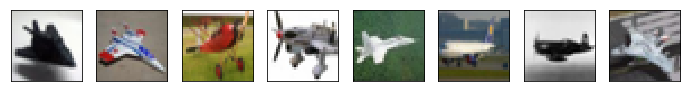automobile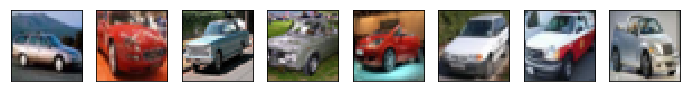bird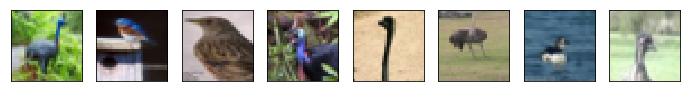cat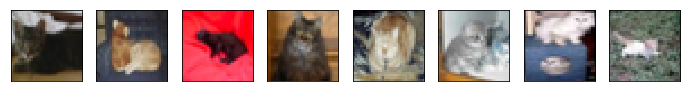deer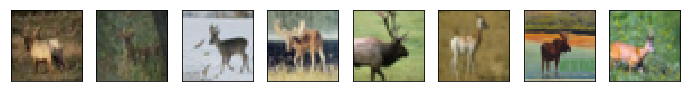dog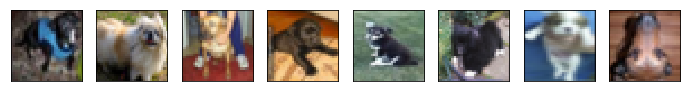frog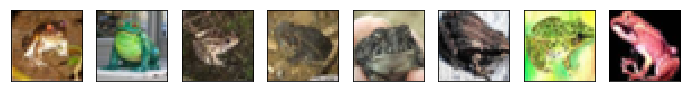horse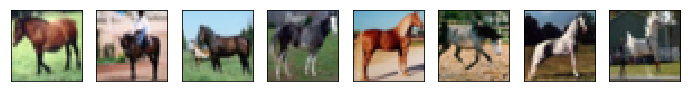shiptruck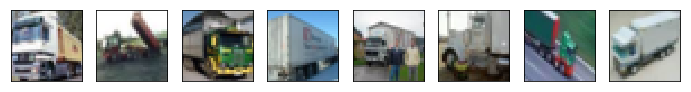### Data Preprocessing and Image Augmentation¶

First let's use Keras's pre-built onehot encoder to define a convenient function for one-hot encoding our class labels later on during model training and evaluation.

In :
def get_onehot(y_data, num_classes=10):
return K.utils.to_categorical(y_data, num_classes)


Next we'll implement image augmentation, which is a technique for improving the generalization of our models by applying randomized image transformations (such as shifts, rotations, shears, zooms, and flips) to the original dataset. This helps force the model to learn feature maps that are more robust and relevant. We are essentially creating a larger training dataset from a smaller one. For excellent introductory articles on this topic, see these posts by Bharath Raj and Ryan Allred.

For our WRN model, it turns out that image augmentation is essential, otherwise our network basically just memorizes our training set (training accuracy reaches 100%) and yields poor generalization to the test set.

Fortunately Keras has built-in tools for applying image augmentation, with examples available here.

We'll base our image augmentation on the scheme recommended by Sergey Zagoruyko and Nikos Komodakis in their seminal paper on Wide ResNets (WRNs). Quoting their paper:

"For data augmentation we do horizontal flips and take random crops from image padded by 4 pixels on each side, filling missing pixels with reflections of original image. We don’t use heavy data augmentation... Unless mentioned otherwise, for CIFAR we follow the image preprocessing of  with ZCA whitening."

Note that ZCA whitening is a linear algebra transformation, similar to principal component analysis (PCA), that reduces pixel redundancy to better highlight the key structures and features in the image. We'll use ZCA whitening to preprocess and standardize our data. We'll also look at how it compares to typical data standardization (mean/std) for our particular WRN model.

Below we create generators for augmented images that also apply ZCA whitening and feature normalization.

In :
# augmented image generator based on scheme
# recommended by Zagoruyko and Komodakis
train_datagen_1 = ImageDataGenerator(
featurewise_center=True,
samplewise_center=False,
featurewise_std_normalization=False,
samplewise_std_normalization=False,
zca_whitening=True,
zca_epsilon=1e-06,
rotation_range=0.0,
width_shift_range=0.25,
height_shift_range=0.25,
brightness_range=None,
shear_range=0.0,
zoom_range=0.0,
channel_shift_range=0.0,
fill_mode='reflect',
cval=0.0,
horizontal_flip=True,
vertical_flip=False,
rescale=None,
preprocessing_function=None,
data_format='channels_last',
validation_split=0.0)

# an alternative image augmentation scheme
# using zooms, rotations, and shears
train_datagen_2 = ImageDataGenerator(
featurewise_center=True,
samplewise_center=False,
featurewise_std_normalization=False,
samplewise_std_normalization=False,
zca_whitening=True,
zca_epsilon=1e-06,
rotation_range=15.0,
width_shift_range=0.1,
height_shift_range=0.1,
brightness_range=None,
shear_range=0.2,
zoom_range=0.2,
channel_shift_range=0.0,
fill_mode='nearest',
cval=0.0,
horizontal_flip=True,
vertical_flip=False,
rescale=None,
preprocessing_function=None,
data_format='channels_last',
validation_split=0.0)

# for the test set, apply ZCA and centering only
test_datagen = ImageDataGenerator(
featurewise_center=True,
zca_whitening=True,
data_format='channels_last')

# data normalization and ZCA whitening require our
# generators to first be fit on our training data
train_datagen_1.fit(np.vstack((X_train, X_val)))
train_datagen_2.fit(np.vstack((X_train, X_val)))
test_datagen.fit(np.vstack((X_train, X_val)))


#### Visualizing the ImageDataGenerator Output¶

Let's have a look at what our image augmentation schemes produce for a sample input image. To make this easier to see, I've defined two new generators with the ZCA whitening transformation removed.

In :
# sample image to augment
x_temp = X_train.reshape(1, 32, 32, 3)

# recreate the generators but without ZCA whitening
demo_datagen_1 = ImageDataGenerator(
rotation_range=0.0,
width_shift_range=0.25,
height_shift_range=0.25,
fill_mode='reflect',
horizontal_flip=True,
data_format='channels_last')

demo_datagen_2 = ImageDataGenerator(
rotation_range=15.0,
width_shift_range=0.1,
height_shift_range=0.1,
shear_range=0.2,
zoom_range=0.2,
fill_mode='nearest',
horizontal_flip=True,
data_format='channels_last')

In :
# plot examples from scheme 1
print('\nData Augmentation Scheme 1:')
i=0
figure = plt.figure(figsize=(14, 8))
for batch in demo_datagen_1.flow(x_temp, batch_size=1):
# display the images
ax = figure.add_subplot(4, 5, i + 1, xticks=[], yticks=[])
img = np.squeeze(batch).astype('uint8')
ax.imshow(img, interpolation='nearest')
i+=1
if i==20:  # otherwise the generator would continue indefinitely
break

Data Augmentation Scheme 1: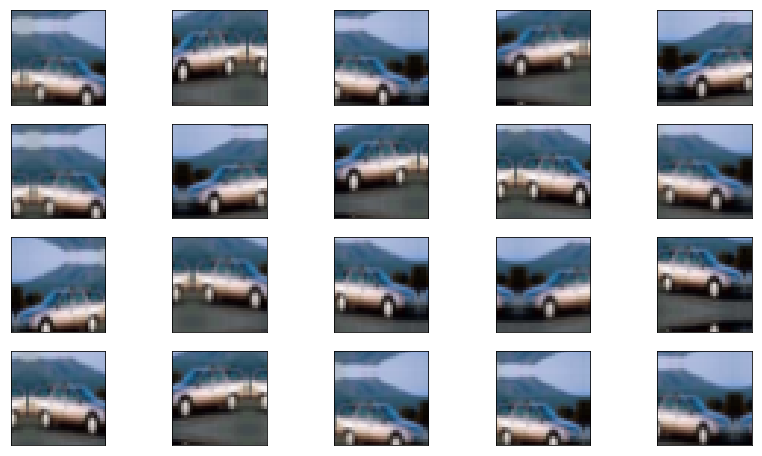In :
# plot examples from scheme 2
print('\nData Augmentation Scheme 2:')
i=0
figure = plt.figure(figsize=(14, 8))
for batch in demo_datagen_2.flow(x_temp, batch_size=1):
# display the images
ax = figure.add_subplot(4, 5, i + 1, xticks=[], yticks=[])
img = np.squeeze(batch).astype('uint8')
ax.imshow(img, interpolation='nearest')
i+=1
if i==20:  # otherwise the generator would continue indefinitely
break

Data Augmentation Scheme 2: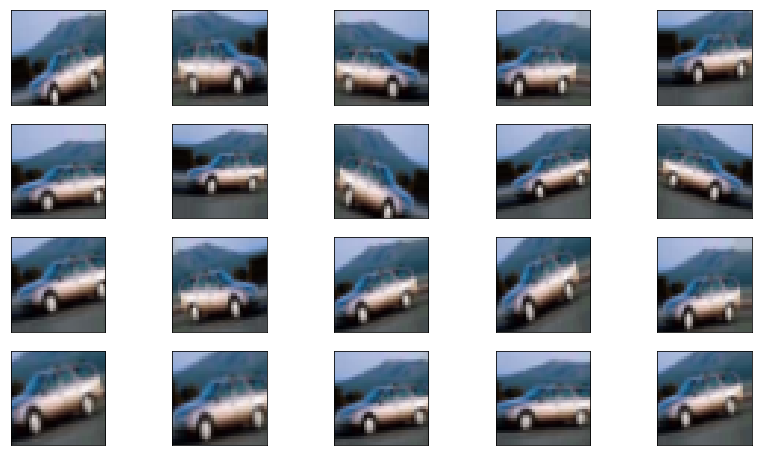## 2) Building a Simplified VGG Network with the Keras Sequential API¶

We'll first turn our attention to the famous VGG (Visual Geometry Group) architecture, which was highly influential in its approach to convolutional networks for image recognition. Compared to earlier architectures, VGG was remarkable in both its simplicity and its layer depth. It is based on a heirarchical representation of visual data that makes it possible for smaller convolutional filter sizes (e.g. 3x3 pixels) to capture rich feature information so long as the network is sufficiently deep. To better appreciate VGG's place in the evolution of state-of-the-art networks for image recognition, I recommend reading this article on the history of neural networks by Prof. Eugenio Culurciello at Purdue University.

The core design rules of VGG network construction are as follows:

1. For a given output feature map size (e.g. 32x32 or 16x16), you use the same number filters (channels) for each convolution layer.
2. Each time the feature map size is halved (e.g. by maxpooling with a stride of 2), you double the number of filters (channels) to maintain the same "time complexity per layer".
3. You end with a global average pooling layer followed by fully-connected (dense) layers and softmax.

A table summarizing various VGG models, taken from the original research paper, is shown below, where convN-K refers to a convolutional layer having filter size NxN pixels and K filter channels.The relative simplicity of VGG makes it an excellent first example for showing how we can build such networks in Keras. On the other hand, its large number of trainable parameters makes it computationally expensive. Hence we'll implement a simplified version of VGG that is similar to configuration B (see table above) but has one less convolution block and only one fully-connected dense layer at the output.

### Building the VGG Model¶

For architectures such as VGG where all network layers are arranged in serial, we can use Keras' Sequential API for model construction. We first create an instance of the Sequential() model object, and then chronologically add layers. The pre-built network layers we'll be using include Conv2D, BatchNormalization, MaxPooling2D, and Dense. A Flatten layer is used to concatenate and reshape all feature maps into a single vector prior to applying the fully-connected layer. The handy summary() method allows us to view details about the model, including the number of trainable and non-trainable parameters.

In :
def build_SVGG():

model = K.Sequential()

# 1. First Conv block
kernel_size=3,
activation='relu',
input_shape=(32, 32, 3)))
kernel_size=3,
activation='relu'))

# 2. Second Conv block
kernel_size=3,
activation='relu'))
kernel_size=3,
activation='relu'))

# 3. Third Conv block
kernel_size=3,
activation='relu'))
kernel_size=3,
activation='relu'))

# 4. Dense layer with dropout applied to flattened data

return model

# for inspection purposes
model_SVGG = build_SVGG()
model_SVGG.summary()

_________________________________________________________________
Layer (type)                 Output Shape              Param #
=================================================================
conv2d_1 (Conv2D)            (None, 32, 32, 64)        1792
_________________________________________________________________
batch_normalization_1 (Batch (None, 32, 32, 64)        256
_________________________________________________________________
conv2d_2 (Conv2D)            (None, 32, 32, 64)        36928
_________________________________________________________________
batch_normalization_2 (Batch (None, 32, 32, 64)        256
_________________________________________________________________
max_pooling2d_1 (MaxPooling2 (None, 16, 16, 64)        0
_________________________________________________________________
conv2d_3 (Conv2D)            (None, 16, 16, 128)       73856
_________________________________________________________________
batch_normalization_3 (Batch (None, 16, 16, 128)       512
_________________________________________________________________
conv2d_4 (Conv2D)            (None, 16, 16, 128)       147584
_________________________________________________________________
batch_normalization_4 (Batch (None, 16, 16, 128)       512
_________________________________________________________________
max_pooling2d_2 (MaxPooling2 (None, 8, 8, 128)         0
_________________________________________________________________
conv2d_5 (Conv2D)            (None, 8, 8, 256)         295168
_________________________________________________________________
batch_normalization_5 (Batch (None, 8, 8, 256)         1024
_________________________________________________________________
conv2d_6 (Conv2D)            (None, 8, 8, 256)         590080
_________________________________________________________________
batch_normalization_6 (Batch (None, 8, 8, 256)         1024
_________________________________________________________________
max_pooling2d_3 (MaxPooling2 (None, 4, 4, 256)         0
_________________________________________________________________
flatten_1 (Flatten)          (None, 4096)              0
_________________________________________________________________
dense_1 (Dense)              (None, 1024)              4195328
_________________________________________________________________
dropout_1 (Dropout)          (None, 1024)              0
_________________________________________________________________
dense_2 (Dense)              (None, 10)                10250
=================================================================
Total params: 5,354,570
Trainable params: 5,352,778
Non-trainable params: 1,792
_________________________________________________________________


We see that this simplified VGG model has roughly 5.3 million parameters.

### Model Compilation and Helper Functions¶

Before we train our model, we need to compile it using the compile() method. It is at this time that we specify:

1. the loss function to be used during optimization,
2. the optimization technique, and
3. the metrics to be used for evaluating model performance.

For example, to train a model using categorical cross-entropy loss with optimization via stochastic gradient descent while tracking accuracy as the performance metric, we use:

model_SVGG.compile(loss='categorical_crossentropy', optimizer='sgd', metrics=['accuracy'])

There are a few other things we need to know about before proceeding. One is about the use of callbacks. Callbacks allow us to apply functions or access model parameters during the training process. For example, we can use callbacks to access the model weights after each training iteration and save these to file if they result in a better minimization of the loss function than previous iterations. We can also use callbacks to generate TensorBoard files for viewing a model's performance and for detailed visualization of its computational graph. For more about callbacks, see the Keras documentation.

Finally, one typically trains, evaluates, and utilizes Keras models by calling the fit(), evaluate(), and predict() methods respectively. In this case, however, when working with data generators for image augmentation, we need to call slightly different methods, namely fit_generator(), evaluate_generator(), and predict_generator(), where our train and test set data generators are given as arguments. Note that we cannot mix these sets of methods: even though we don't use image augmentation on the test set, we cannot simply standardize the test data and call predict() after training a model with fit_generator(); doing so will yield erroneous results, hence why we use a generator for the test set.

Calling fit() or fit_generator() on a model object returns a history object that we can use to plot training and validation accuracy as a function of training epochs, which is great for assessing how well the model converges and its generalization (e.g. bias-variance tradeoff).

Below we define some helper functions that make it easier for us to train and evaluate our Keras models with fewer lines of repeated code:

In :
# perform all tasks related to model training
def compile_and_train(model,
MODEL_NAME,
X_train_data,
y_train_data,
X_val_data,
y_val_data,
train_generator,
test_generator,
BATCH_SIZE,
EPOCHS,
optimizer='rmsprop',
lr_schedule_fn=None,
starting_epoch=0):

# directory setup for saving model files
MODEL_DIR = '/home/rpm/Dropbox/Keras-Devs/models/CIFAR-10/'

DIR = os.path.join(
MODEL_DIR,
MODEL_NAME,
datetime.now().strftime('%Y%m%d-%H%M%S'))

if not os.path.exists(DIR):
os.makedirs(DIR)

LOG_DIR = os.path.join(DIR, 'logs')

# checkpointer callback for saving best weights
WEIGHT_DIR = os.path.join(DIR, 'best_weights.hdf5')
checkpointer_cb = K.callbacks.ModelCheckpoint(
filepath=WEIGHT_DIR,
verbose=1,
save_best_only=True)

# callback to save tensorboard logs
tensorboard_cb = TensorBoard(
log_dir=LOG_DIR,
histogram_freq=0,
batch_size=BATCH_SIZE,
write_graph=True,
write_images=False)

print('To view graph generated by TensorBoard,')
print('copy+paste the following into terminal:')
print('\ntensorboard --logdir=%s' % LOG_DIR)
print('\n')

# special callbacks for a scheduled learning rate
if lr_schedule_fn is not None:

# learning rate scheduler
lr_schedule_cb =K.callbacks.LearningRateScheduler(
lr_schedule, verbose=0)

# custom history callback
class LossHistory(K.callbacks.Callback):
def on_train_begin(self, logs={}):
self.losses = []
self.lr = []

def on_epoch_end(self, batch, logs={}):
self.losses.append(logs.get('loss'))
self.lr.append(lr_schedule(len(self.losses)))

loss_and_lr_history_cb = LossHistory()

callback_list = [checkpointer_cb,
tensorboard_cb,
lr_schedule_cb,
loss_and_lr_history_cb]
else:

callback_list = [checkpointer_cb,
tensorboard_cb]

# compile the model
model.compile(loss='categorical_crossentropy',
optimizer=optimizer,
metrics=['accuracy'])

# one-hot encode labels
y_train_data_onehot = get_onehot(y_train_data)
y_val_data_onehot = get_onehot(y_val_data)

# prepare data generators
train_gen = train_generator.flow(
X_train_data,
y_train_data_onehot,
batch_size=BATCH_SIZE)

val_gen = test_generator.flow(
X_val_data,
y_val_data_onehot,
batch_size=BATCH_SIZE)

# train the model
history= model.fit_generator(
generator=train_gen,
steps_per_epoch=len(X_train_data)/BATCH_SIZE,
epochs=EPOCHS,
verbose=1,
callbacks=callback_list,
validation_data=val_gen,
validation_steps=len(X_val_data)/BATCH_SIZE,
class_weight=None,
max_queue_size=10,
workers=1,
use_multiprocessing=False,
shuffle=True,
initial_epoch=starting_epoch)

# save trained model (after final epoch)
model.save(os.path.join(DIR, 'model_WRN.h5'))

if lr_schedule_fn is not None:
return (history, DIR, loss_and_lr_history_cb)
else:
return (history, DIR)

In :
# function for plotting metrics versus
# training epoch from a history object
def plot_histories(history_):

# summarize history for accuracy
plt.plot(history_.history['acc'])
plt.plot(history_.history['val_acc'])
plt.title('model accuracy')
plt.ylabel('accuracy')
plt.xlabel('epoch')
plt.legend(['training', 'validation'], loc='upper left')
plt.show()

# summarize history for loss
plt.plot(history_.history['loss'])
plt.plot(history_.history['val_loss'])
plt.title('model loss')
plt.ylabel('loss')
plt.xlabel('epoch')
plt.legend(['training', 'validation'], loc='upper left')
plt.show()

return None

# helper function for evaluating performance
def evaluate_performance(model_obj,
model_dir,
X_test_data,
y_test_data,
test_generator,
model_hist=None,
model_lr_hist=None):

# plot accuracies versus epoch
if model_hist is not None:
print('\nPlotting Training History:')
plot_histories(model_hist)

# plot learning rate if scheduled
if model_lr_hist is not None:
print('\nPlotting Learning Rate Schedule:')

plt.plot(model_lr_hist.lr)
plt.ylabel('Learning Rate')
plt.xlabel('Epoch')
plt.show()

plt.plot(model_lr_hist.losses)
plt.ylabel('Loss')
plt.xlabel('Epoch')
plt.show()

# one-hot encoding
y_test_data_onehot = get_onehot(y_test_data)

# prepare data generator
test_gen = test_generator.flow(
X_test_data,
y_test_data_onehot,
batch_size=1)

# evaluate with End-Of-Training weights
print('\nComputing EOT Score...')
score_EOT = model_obj.evaluate_generator(
generator=test_gen,
steps=len(y_test_data),
max_queue_size=10,
workers=1,
use_multiprocessing=False,
verbose=1)
print('Test Accuracy with EOT Weights:', score_EOT)

# evaluate with Best Weights
os.path.join(model_dir, 'best_weights.hdf5'))

print('\nComputing Best Weights Score...')
score_BW = model_obj.evaluate_generator(
generator=test_gen,
steps=len(y_test_data),
max_queue_size=10,
workers=1,
use_multiprocessing=False,
verbose=1)
print('Test Accuracy with Best Weights:', score_BW)

scores = (score_EOT, score_BW)

return scores


### Model Training¶

With our handy helper functions, we can now build and train the simplified VGG network in a few short chunks of code. Note that I'm substituting the test set as the validation set here, so we can see how the test accuracy evolves with model training --- I can do this here because in no way is the test set being used to update the model weights during training or to select hyperparameters. Although I made a validation set split earlier out of habit, here I'm training the model on the full 50000-sample training set.

In :
# reset the tensorflow graph
K.backend.clear_session()

# construct model
model_SVGG = build_SVGG()

# initiate training
this_name = 'SVGG_AugScheme1_FullTrainSet'

(this_history, this_dir) = compile_and_train(
model=model_SVGG,
MODEL_NAME=this_name,
X_train_data=np.vstack((X_train, X_val)),
y_train_data=np.hstack((y_train, y_val)),
X_val_data=X_test,
y_val_data=y_test,
train_generator=train_datagen_1,
test_generator=test_datagen,
BATCH_SIZE=128,
EPOCHS=400,
optimizer='rmsprop',
lr_schedule_fn=None)

To view graph generated by TensorBoard,
copy+paste the following into terminal:

tensorboard --logdir=/home/rpm/Dropbox/Keras-Devs/models/CIFAR-10/SVGG_AugScheme1_FullTrainSet/20180917-212553/logs

Epoch 1/400
391/390 [==============================] - 94s 241ms/step - loss: 2.4747 - acc: 0.3377 - val_loss: 2.0399 - val_acc: 0.3977

Epoch 00001: val_loss improved from inf to 2.03992, saving model to /home/rpm/Dropbox/Keras-Devs/models/CIFAR-10/SVGG_AugScheme1_FullTrainSet/20180917-212553/best_weights.hdf5
Epoch 2/400
391/390 [==============================] - 94s 241ms/step - loss: 1.6176 - acc: 0.5055 - val_loss: 1.3853 - val_acc: 0.5288

Epoch 00002: val_loss improved from 2.03992 to 1.38532, saving model to /home/rpm/Dropbox/Keras-Devs/models/CIFAR-10/SVGG_AugScheme1_FullTrainSet/20180917-212553/best_weights.hdf5
Epoch 3/400
391/390 [==============================] - 103s 263ms/step - loss: 1.3972 - acc: 0.5690 - val_loss: 1.9149 - val_acc: 0.4996

Epoch 00003: val_loss did not improve from 1.38532
Epoch 4/400
391/390 [==============================] - 100s 256ms/step - loss: 1.2094 - acc: 0.6155 - val_loss: 1.2153 - val_acc: 0.6067

.
.
.

Epoch 399/400
391/390 [==============================] - 93s 237ms/step - loss: 0.5183 - acc: 0.8951 - val_loss: 0.8853 - val_acc: 0.8774

Epoch 00399: val_loss did not improve from 0.46327
Epoch 400/400
391/390 [==============================] - 93s 239ms/step - loss: 0.5361 - acc: 0.8944 - val_loss: 1.0267 - val_acc: 0.8568

Epoch 00400: val_loss did not improve from 0.46327


### Model Evaluation¶

Now let's run our evaluation helper function to see how this model did:

In :
# function for evaluating performance
this_score = evaluate_performance(
model_obj=model_SVGG,
model_dir=this_dir,
X_test_data=X_test,
y_test_data=y_test,
test_generator=test_datagen,
model_hist=this_history,
model_lr_hist=None)

Plotting Training History: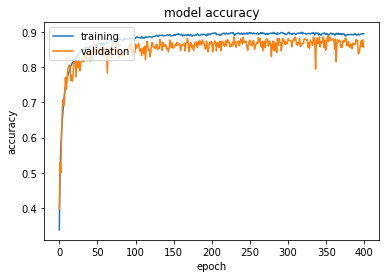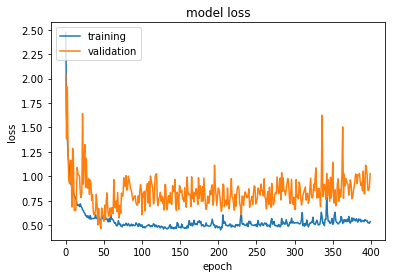Computing EOT Score...
10000/10000 [==============================] - 29s 3ms/step
Test Accuracy with EOT Weights: 0.8568

Computing Best Weights Score...
10000/10000 [==============================] - 27s 3ms/step
Test Accuracy with Best Weights: 0.8637


Performance on the training set saturates fairly quickly (around epoch 100) whereas the loss function for the valdiation set appears to reach an optimum near 50 epochs and then fluctuates significantly. To obtain better performance, we could try a different optimizer or specify a learning rate schedule. For now, we'll note 86.4% as the test accuracy obtained by this model.

### View Sample Predictions¶

Let's view a random sample of the images our trained model has classified correctly or incorrectly. The following code accomplishes this.

In :
# generate the predictions
test_generator = test_datagen.flow(X_test,
get_onehot(y_test),
batch_size=1)

probas = model_SVGG.predict_generator(test_generator,
steps=len(y_test))
preds_SVGG = np.argmax(probas, axis=1)

# function for viewing predictions
def view_predictions(model_, preds_, condition='None'):
if condition is None:
print('\n**Displaying 20 Randomly-Selected Predictions**')
inds = np.arange(len(preds_))
elif condition is 'Correct':
print('\n**Displaying Correct Predictions**')
inds = np.arange(len(preds_))[preds_ == y_test]
elif condition is 'Incorrect':
print('\n**Displaying Incorrect Predictions**')
inds = np.arange(len(preds_))[preds_ != y_test]
else:
raise ValueError(
'condition must be: "None", "Correct" or "Incorrect"')

# plot 20 randomly-chosen samples
figure = plt.figure(figsize=(14, 8))
for i, index in enumerate(np.random.choice(inds,
size=20,
replace=False)):

# display the images
ax = figure.add_subplot(4, 5, i + 1, xticks=[], yticks=[])
ax.imshow(X_test[index].astype('uint8'), interpolation='None')

# from one-hot encoded back to integer label
pred_int = preds_[index]
true_int = y_test[index]

# set title: Prediction (True Class)
ax.set_title("{} ({})".format(
label_dict[pred_int],
label_dict[true_int]),
color=("green" if pred_int == true_int else "red"))

return None

# correct predictions
view_predictions(model_SVGG, preds_SVGG, condition='Correct')

**Displaying Correct Predictions**